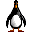Pixels to Centimeters
Vnik from Colombia  [13 posts]
14 year
 I want to know the position of some robots; I have a static overhead camera and use RoboRealm to acquire position. This position is in Pixels, it gives me for example coordinates: X=180, Y= 250.  but they are in pixels and I want to know it's equivalency to centimeters. Is there a formula to know how many centimeters are one pixel?? I know that I need the camera parameters and I obtained them with the Matlab Camera Calibration Toolbox. Thanks a lot for any help.Anonymous 14 year
 Vnik, The technqiue used will depend on the precision that you need. For example, if you have a rectified image (i.e. on that any barrel distortion or perspective distortion is removed) you can simply place a ruler on the floor and count how many pixels it takes for 30 cm. Your conversion factor would then be factor = number_of_pixels / 30 and your pixel to cm conversion would be distance = pixels * factor which would give you a rough estimate of distance. The big issue is that if the camera ever moves either closer or further away the factor will be incorrect and therefore give you wrong results. So this only works for a stationary camera and scene. If the camera and scene are moving you will either need stereo to calculate distance, another sensor like Lidar or known objects in the scene that are a known size (in which to do on-the-fly calibration). STeven.Vnik from Colombia  [13 posts] 14 year
 STeven, This is what I understood: I put a 90cm rule on the floor. I use the RoboRealm calculate distance module to know the pixels (a program I found on the forum that uses a START X, START Y, END X, END Y and you just click on the screen and result is saved on Lenght variable). After this process I have this: Lenght is approximately: 254 pixels (I click on what looks the ruler' origin and then ruler end, length is +- 254 pixels). So if the ruler have 90cm: Factor = 254pixels/90cm = 2,82 pixels/cm So if for example I want to know at how many centimeters is placed a robot that is at  X:93 pixels, Y:123 pixels I should use the factor and said that the robot is at: X:93 pixels * 2,82 pixels/cm = 262,26 pixels^2/cm Y:123 pixels * 2,82 pixels/cm =  346,86 pixels^2/cm Final result shouldn't be only in centimeters?Anonymous 14 year
 Vnik, Sorry about that ... I guess little sleep + simple math = bad idea! The formula is inverted. Either switch the factor around or divide by the factor. So the correct formula is Factor = 90cm/254pixels = 0,3543307cm/pixel X:93 pixels * 0,3543307cm/pixel = 32,96 cm Y:123 pixels * 0,3543307cm/pixel =  43,58 cm Now note that this is approximate as we have assumed that the image has been undistorted AND that each pixel/CCD sensor is perfectly square. If the CCD is not perfectly square your X or Y may need adjustment for that. You can test to see if the current formula gets you the results you need by actually measuring the physical setup to see if it comes close. Also note that the longer the measurement (i.e. 90cm) the better as it is hard to count subpixels. But again, 90 may be long enough for your project. We will probably add this to the calculate distance module as it seems like a nice extension to that. I.e. you would be able to enter in the two measurements and it would auto scale into cm. Thanks, STeven.

 This forum thread has been closed due to inactivity (more than 4 months) or number of replies (more than 50 messages). Please start a New Post and enter a new forum thread with the appropriate title.